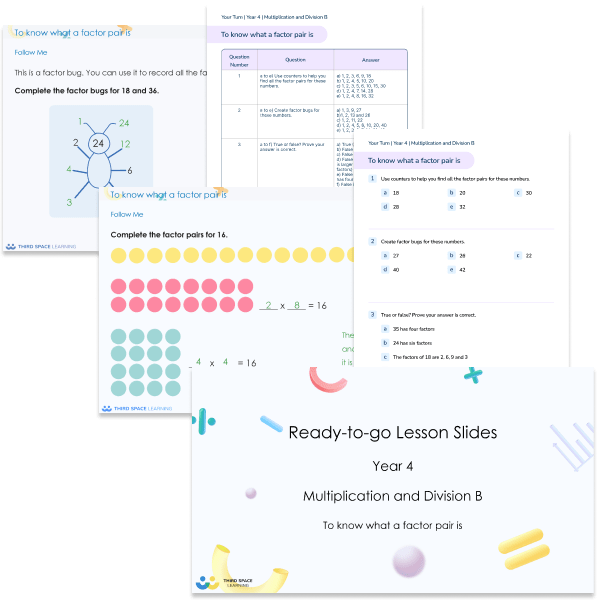# Ready-to-go Lessons Year 4 Multiplication and Division B

This multiplication and division B Ready-to-go lessons pack for Year 4 includes 15 PowerPoints with matching worksheets ready for you to use with a class. These resources follow a mastery approach to mathematics teaching. The PowerPoints are fully editable with detailed notes for every teaching slide. Each associated worksheet is divided into three parts with a range of activities to complete across the learning area being covered.

This Year 4 multiplication and division B pack contains 15 fully editable PowerPoints and 15 PDF worksheets.

These slides and worksheets cover:

• Understanding and using factor pairs
• Multiplying and dividing by 10 and 100
• Related calculations
• Informal methods of multiplication
• Multiplying 2-digit and 3-digit numbers by 1 digit numbers
• Partitioning to divide
• Dividing 2-digit and 3-digit numbers by 1-digit numbers
• Correspondence problems
• Efficient methods of multiplication

Year 4

Multiplication, Division